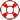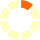Standard:

Description:

Standard:

Description:

Standard:

Description:

Standard:

Description:

Standard:

# 7.11.B

Description:

## determine if the given value(s) make(s) one-variable, two-step equations and inequalities true; and7th Grade Math - Represent & Solve Inequalities Lesson

Sometimes solutions to inequalities are represented on a number line.
To use a number line representation, first, place an open circle on the tick mark at the maximum value (for less than) or minimum value (for greater than).
Then, extend an arrow from the circle to the left or right, depending on if the solutions are less than or greater than that value, respectively.
If the inequality is ≥ or ≤, use a closed circle instead of an open circle.# Processing Request...